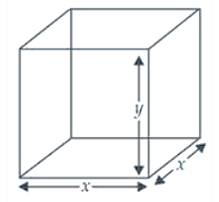Deepak Scored 45->99%ile with Bounce Back Crack Course. You can do it too!

# An open box with square base is to be made of a given quantity

Question:

An open box with square base is to be made of a given quantity of card board of area c2. Show that the maximum volume of the box is c3/ 6√3 cubic units.

Solution:

Let x be the length of the side of the square base of the cubical open box and y be its height.

So, the surface area of the open box$c^{2}=x^{2}+4 x y \Rightarrow y=\frac{c^{2}-x^{2}}{4 x}$   $\ldots(i)$

Now volume of the box, $\mathrm{V}=x \times x \times y$

$V=x^{2} y$

$\mathrm{V}=x^{2}\left(\frac{c^{2}-x^{2}}{4 x}\right)$

$\Rightarrow \quad \mathrm{V}=\frac{1}{4}\left(c^{2} x-x^{3}\right)$

Differentiating both sides w.r.t. $x$, we get

$\frac{d \mathrm{~V}}{d x}=\frac{1}{4}\left(c^{2}-3 x^{2}\right)$ .......(ii)

For local maxima and local minima, $\frac{d V}{d x}=0$

$\therefore \quad \frac{1}{4}\left(c^{2}-3 x^{2}\right)=0 \Rightarrow c^{2}-3 x^{2}=0$

$\Rightarrow \quad x^{2}=\frac{c^{2}}{3}$

$\therefore \quad x=\sqrt{\frac{c^{2}}{3}}=\frac{c}{\sqrt{3}}$

Now again differentiating eq. (ii) w.r.t. $x$, we get

$\frac{d^{2} \mathrm{~V}}{d x^{2}}=\frac{1}{4}(-6 x)=\frac{-3}{2} \cdot \frac{c}{\sqrt{3}}<0$ (maxima)

Volume of the cubical box $(\mathrm{V})=x^{2} y$

$=x^{2}\left(\frac{c^{2}-x^{2}}{4 x}\right)=\frac{c}{\sqrt{3}}\left[\frac{c^{2}-\frac{c^{2}}{3}}{4}\right]=\frac{c}{\sqrt{3}} \times \frac{2 c^{2}}{3 \times 4}=\frac{c^{3}}{6 \sqrt{3}}$

Therefore, the maximum volume of the open box is

$\frac{c^{3}}{6 \sqrt{3}}$ cubic units.